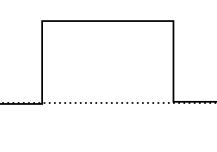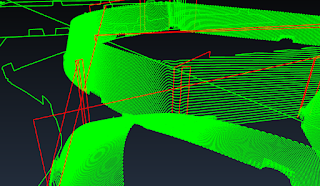## Posts

Showing posts from April, 2018

### How to get sinusoidal s-curve for a stepper motorAfter some experimentation, and delving a bit into the math of motion, I realized there can be a significant difference on the motion of a machine by just making small changes.

A common and popular approach to smooth one axis of motion is to use a trapezoidal speed pattern, where acceleration and deceleration are uniformly accelerated movements (aka constant acceleration motion) separated by a portion happening at cruising speed.

The problem comes with the fact that, while that approach is simple to understand and to code, it does contain sudden changes in the acceleration, that show as spikes on the acceleration derivative, called jerk. These sudden changes in the acceleration translate into undesired vibration in our machines.

Most of that can be eliminated by selecting a motion pattern that does not contain sudden changes of acceleration. If we chose a mathematical function for our speed whose second derivative is continuous, then we reduce system vibration quite a lot. One may thi…

### Moving code from ESP8266 to ESP32A while ago I made a mashup of Dan Royer's code CNC 2 Axis Demo with my own code for trapezoidal motion stepper and servo control for ESP8266.

I assumed porting the code to the ESP32 would be trivial, and that was true for the most part: changes like library name being Wifi.h instead of Wifi8266.h were not a problem. UDP now does not like multicharacter writes but you can use print instead. So far so good.

However, when it came to the interrupt code I was stuck with the stepper interrupt causing an exception sometimes. And to make things weirder, the servo interrupt worked flawlessly (both of them had the IRAM_ATTR directive if you ask me).

Going little by little, I could narrow down the culprit to a floating point operation during the interrupt, that would cause problems sometimes but not always. Browsing around I found this post. Where the solution was simple: do not use floats within the interrupt routines but doubles. The reason was the float calculation would be performed by…

### Servo control on ESP32One of the things that do not work the Arduino way in the ESP32 so far is the PWM generation using analogWrite() command. There is, however, a library to handle PWM signals using timers, so up to 16 PWM signals can be handled.  You can find fine examples like this one.

However, I wanted to use timers in my project and I wanted to have a clearer view of what was going on, so I decided to handle the PWM timing myself. Here you have an example of how to handle a single servo using a timer. Same timer and routine could be extended to handle more output pins in a similar fashion, but I just needed one here.

It is just a simple sketch that moves the servo back and forth using a sin function to get this motion easily, ranging the signal pulse width from 600 to 2200 microseconds.
This is the end result:

All in all, I am impressed with the simplicity and power of the ESP32 and the great Arduino-esp32 code. Kudos to Espressif.

### ESP-32 in UNO formatA few years ago, the IC manufacturer Expressif surprised many of us with their ESP8266 SoC.  A 32- bit processor, running at 80 or 160 MHz with built-in wifi support. It proved to be a very interesting proposal and, eventually, a versión with the same form-factor as the Arduino UNO was made available (which opened compatibility with many available shields).

The same manufacturer developed the next versión, a new and improved SoC this time with Bluetooth and Wifi support, more I/O pins and low-power modes.

I almost forgot, the main reason these chips got successful in the DIY world was that the price was right and a powerful SDK was made available, that eventually led to a group of enthusiasts to create an Arduino-compatible library that made possible to use Arduino code and Arduino IDE to develop with these chips.

A few weeks ago I was contacted to see if a wifi-enabled stepper motor controller board could be done with an ESP. As that is something I had already done the obvious answer…

### Testing sinusoidal S-curvesMy main goal here was to keep a simple mechanism to be easy to calculate and able to be included into 8-bit firmware like Marlin. Which by the way already has support for s-curves for quite a while (at least on this mod for Ultimaker).

So the goal is to have a function f(t) that will map the speed at each given moment but in a way that the maximum speed will be reached at the same time as it would using a uniform acceleration, so the rest of the math and motion planning is not affected. In doing so, the peak acceleration is going to be higher than that when using uniform acceleration. However, if that is a problem it is ok to reduce the maximum acceleration so the system can work flawlessly.

I have tested a couple of functions, both based on sinusoidal functions, the former is:
f(t)=V*(1-cos(PI*(t/t1))/2 (where V is max speed and t1 marks the end of the S-curve ramping up). It is the dotted-green curve below. The shape of the acceleration for this speed pattern is the dotted-pink curv…

### Sinusoidal functions for s-curve motionWhile trapezoidal speed motion pattern is good and well. It is well known that the sudden changes in acceleration it requires, also known as jerk (jerk being the time derivative of the acceleration) is the cause of trouble in many machines.

That first model can be improved with another acceleration shape that will remove the sudden changes, thus improving the system performance.

However, will there are no sharp edges on the acceleration values, there is still a couple of points where there is a not so smooth change. So the next idea, while still within the use of a sinusoidal acceleration profiles is to change to this other acceleration profile:
This new curve has smoother transitions from zero to any other value, thus no more problems with the jerk. However, we still can think of a combination of this latter case and the initial one, where corners are smoothed out using a sinusoidal: That is what they suggest in this paper
While I was not eager to use any complex math in my dcservo

### Speeding up my CAMA while ago, I created from scratch a CAM software. It has been consistently working ok with a minor problem of occasional wounds in some parts, which I narrowed down to a bad condition.

But solving the math was only part of the problem. A problem my students found too difficult to crack. So I have to provide them a solution (this was for a ball end mill).

The computing time of the algorithm depends on how many calculations are done. And the brute-force approach of checking every single point, edge and triangle for each sample point is just too slow (but works flawlessly).

I have used a dual approach to speed up the code. On one hand, I am selecting only a few elements to be checked for each vertical scan line, that reduces significantly the number of checks to those that are relevant for the current scan line. The second idea is to write multi-threaded code that can handle different scan lines using a different thread, that in turn will run in a different core of the processor if I d…